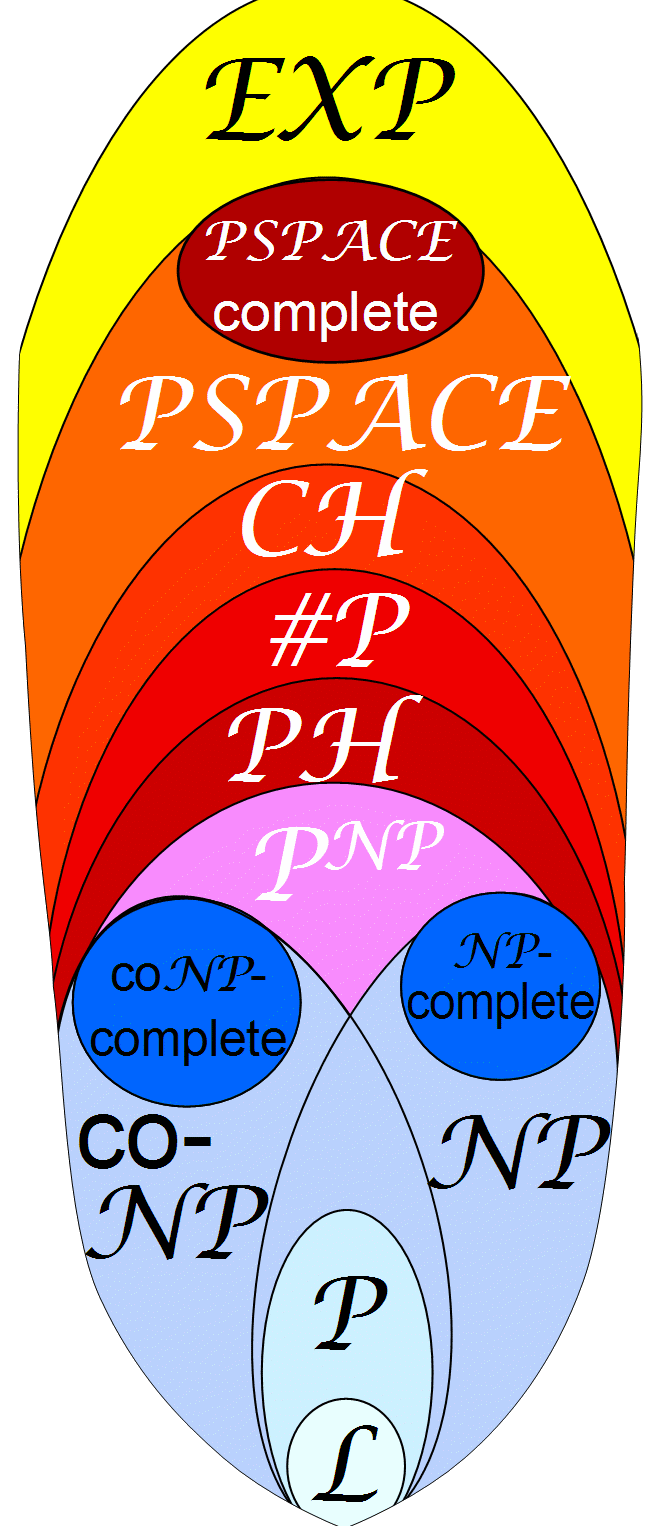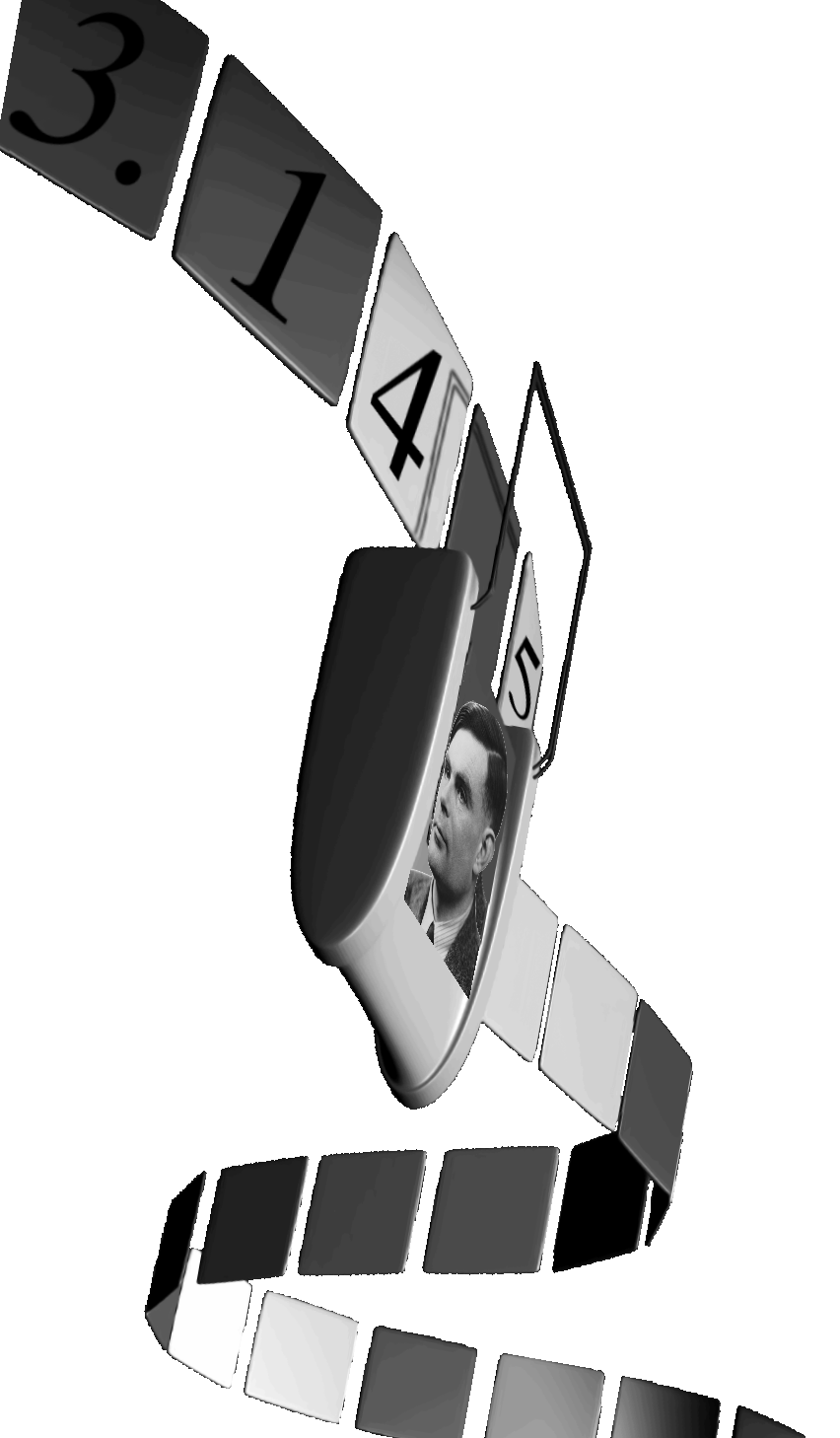## Complexity and Real Computation Lab.The classical theory of computation (models and algorithms, computability and complexity, semantics and specification etc.) is concerned with discrete problems, that is, over bits or integers. We apply, adapt, and newly develop such methods and concepts to the many continuous problems pertaining to and arising in analysis/numerics, algebra, and physics. This includes devising and analyzing rigorous algorithms for calculations involving real (and complex) numbers, functions, and operators; and proving them optimal by relating to famous open conjectures like "P≠NP", both in the bit-cost and in the algebraic model aka Blum-Shub-Smale machine. Promising (e.g. parameterized polynomial-time) algorithms are implemented in an imperative object-oriented programming language, and their practical performance evaluated empirically.

### Complexity and Real Computation Lab.

KAIST, School of Computing
Theory of Computation Group E3-1 3rd floor
291 Daehak-ro (Guseong-dong) Yuseong-gu,
34141 Daejeon, KOREA